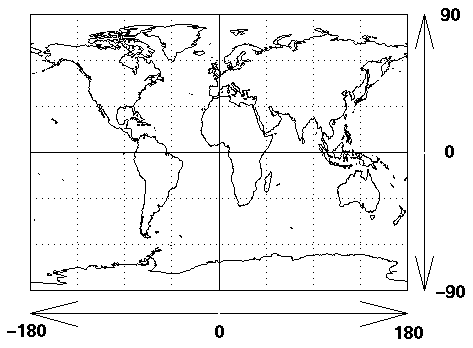# GPS Latitude and Longitude Converter

GPS Latitude / Longitude Coordinate Converter

 Required Data Entry Degrees/Minutes/Seconds DDD MM SS Calculated Results GPS Coordinate DDD MM.MMMM Decimal Degrees DDD.DDDD
 Required Data Entry Decimal Degrees DDD.DDDD Calculated Results GPS Coordinate DDD MM.MMMM Degrees/Minutes/Seconds DDD MM SS
 Required Data Entry GPS Coordinate DDD MM.MMMM Calculated Results Decimal Degrees DDD.DDDD Degrees/Minutes/Seconds DDD MM SSThis converter requires the use of Javascript enabled and capable browsers. It is designed to convert the various distinct methods of co-ordinate nomenclature from one methodology to another. Due to the fact that GPS information is so readily available now for daily weather information, automotive use, aviation, marine and personal use, many companies have set their own standards as to how that information is displayed and disseminated. (Get NAD27 and NAD83 conversion info from NOAA here...) Any method is very accurate in the native form but converting between them can create substantial error. When using a hand held GPS for instance, you may have to use the information and convert it to or from what a GIS map displays. We have created this series of converters to take degrees, minutes and seconds and convert them to decimal degrees or degrees and decimal minutes. In order to establish a point, you MUST have latitude and longitude coordinates in one form or another. If you need to get that information relating to distances between points, use the GPS Latitude and Longitude Distance Calculator. If you need to get that information to a different format, use these converters. (Please keep in mind that this is a conversion utility and NOT an entry for a point. Validity is NOT tested. The minutes and seconds designations are OF ARC and not of time.) The different formats are as follows:

Degrees, minutes and seconds (DDD, MM, SS) is the conventional method of presentation. Remember that in the case of degrees of latitude, the format would be negative or positive DD, while longitude could be negative or positive DDD. There is usually a quadrasphere designation as well, such as N, S, E or W, based on the equator and the prime meridian. For the hemisphere, either North or South, the designation is N or S for latitude. East of the prime meridian is E (positive) and West of the prime meridian is W (negative). Negative numbers (in certain situations, Southern latitude is displayed as negative; if you see a negative latitude, it is South while a negative longitude is West) can also be used to express a quadrasphere designation. In this converter, we do neither since it does not matter; we are ONLY converting numbers. All numbers are assumed to be positive.

Decimal Degrees is displayed as the degrees in normal value, with both minutes and seconds in decimal format, as a degree value. There is ONLY a degree designation. (DDD.DDDD) A maximum of 4 decimal places is adequate.

What is most often known as GPS format (though not all GPS units use it o agree on it...), is displayed as (DDD,MM.MMMM) in which seconds are converted to decimal minutes, as a minute value. Seconds have the value of 0 to 60, with 0 and 60 (usually designated as 0 to 59 and then restarting at 0) being the same value, minutes have the same characteristics and degrees are valid from 0 to 180 and 180 to 0, (0 and 180 do NOT have the same value) both North and South of the equator, and East and West of the prime meridian.

If you are not familiar with latitude and longitude, here is a crash course in navigation. (Probably a poor choice of words...) Lines of latitude and longitude are hypothetical lines on the surface of the Earth. On the Earth, lines of latitude are circles of different size. The longest (largest in diameter) is the equator, whose latitude is zero, while at the poles, at latitudes 90° north and 90° south (or -90°), the circles shrink to a point. On the Earth, lines of constant longitude (meridians) extend from pole to pole, and cross the lines of latitude. Every point on the surface of the Earth has coordinates where a given line of latitude and a give line of longitude intersect (cross). To sum it up, latitude is measured from the equator, with positive values going north (0 to 90) and negative values going south (0 to -90). Longitude is measured from the Prime Meridian (which is the longitude that runs through Greenwich, England), with positive values going east (0 to 180) and negative values going west (0 to -180). So, for example, 65 degrees west longitude, 45 degrees north latitude is -65 degrees longitude, +45 degrees latitude. Now that the designations (and reasons for them) are perfectly clear, here is the set of formulae if you need to do this manually.

Degrees Minutes Seconds to Degrees Minutes.m (GPS)
Degrees = Degrees, Minutes.m = Minutes + (Seconds / 60)

Degrees Minutes.m to Decimal Degrees
.d = M.m / 60, Decimal Degrees = Degrees + .d

There are 60 minutes in a degree and 60 seconds in a minute; 3600 seconds in a degree. There are 360 degrees in a complete circle or sphere but in all longitude and latitude measurements, the total of the degrees is expressed as 2 halves of 180 degrees each.
Version 5.1.4

 Leave us a question or comment on FacebookSearch or Browse Our Site